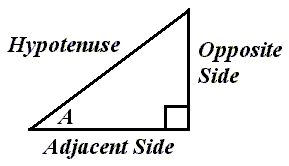# The Right Triangle and the Cosecant FunctionRelative to angle A, this is how the sides of a right triangle would be labeled.

The cosecant of angle A equals the length of the hypotenuse divided by the length of the opposite side.

We could write:

csc(A) = hyp / opp

So, for example, if the length of the hypotenuse was 10 and the length of the opposite side was 6, then we would write:

csc(A) = 10 / 6

csc(A) = 1.6667

Custom Search